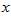## 《高等数学(1)》22春平时作业2-00001

《高等数学(1)》22春平时作业2-00001

------------------------
1.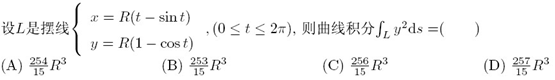A.(A)
B.(B)
C.(C)
D.(D)

2.设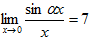，则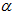的值是 （ 　）
A.0
B.1
C.5
D.7

3.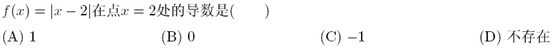A.(A)
B.(B)
C.(C)
D.(D)

4.设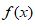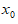可导且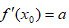，则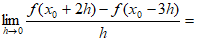（ ）
A.0
B.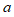C.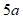D.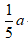5.题面见图片A.A
B.B
C.C
D.D

6.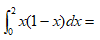（ ）
A.1
B.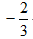C.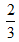D.-1

7.满足方程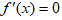，的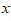是函数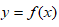的（ ）
A.极大值点
B.极小值点
C.驻点
D.间断点

8.设二元函数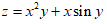，则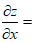（ ）
A.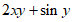B.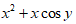C.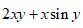D.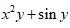9.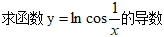（ ）
A.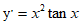B.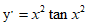C.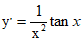D.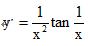10.题面见图片A.A
B.B
C.C
D.D

11.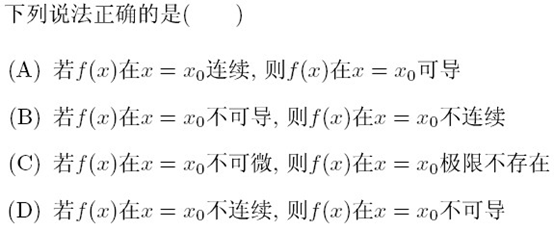A.(A)
B.(B)
C.(C)
D.(D)

12.题面见图片A.A
B.B
C.C
D.D

13.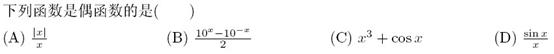A.(A)
B.(B)
C.(C)
D.(D)

14.题面见图片A.A
B.B
C.C
D.D

15.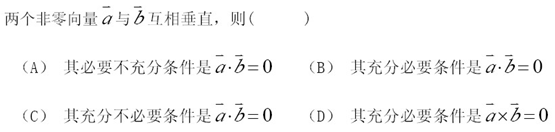A.(A)
B.(B)
C.(C)
D.(D)

16.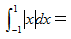（ ）
A.0
B.1
C.2
D.3

17.A.(A)
B.(B)
C.(C)
D.(D)

18.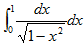等于（ ）
A.0
B.1
C.D.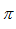19.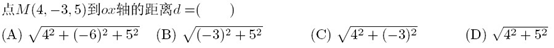A.(A)
B.(B)
C.(C)
D.(D)

20.设函数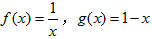，则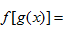（　）
A.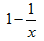B.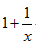C.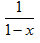D.